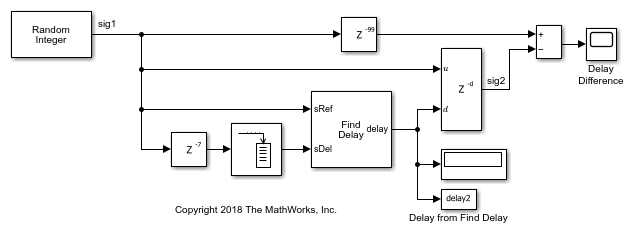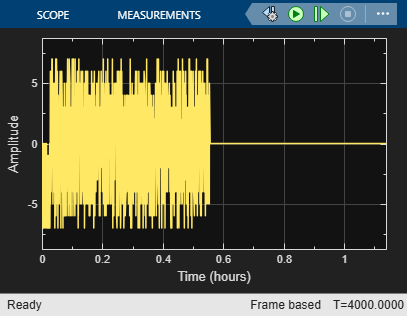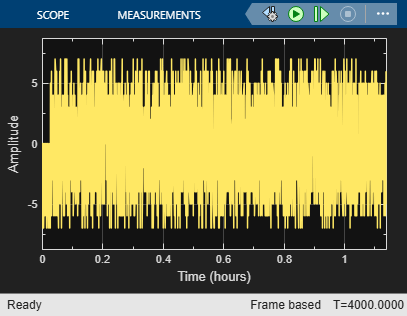# Align Multirate Signals in Simulink

For two signals with different sizes and rates, the delay is resolvable as long as the total delay is less than the reference signal frame length. The `Find Delay` block computes the delay between the mutlirate signals. The signals are delayed and aligned by using `Delay` blocks. The buffer output size is adjusted to show resolvable and unresolvable delays.

### Model Structure

The model uses parallel signal paths to compute and compare the number of samples of delay between two signals, `sig1` and `sig2`.

• In the upper path a fixed delay of 99 samples, which equals 7 plus the buffer value, is added to the reference signal.

• In the lower path, the Find Delay block computes the delay between the two signals. That amount of delay is applied to the undelayed signal, `sig1`, using the Delay (Simulink) block.

Random integer data provides a data stream of 100 sample frames that serves as a reference signal, `sig1`, and as a delay signal, `sig2`. The delay between the reference signal and the delay signal is resolvable as long as the buffered signal length plus the discrete delay length are not greater than the frame length of the reference signal.

When the model runs the delay computed by the `Find Delay` block is displayed. The difference between the reference signal with a fixed delay added and the computed delay added is plotted. When the plotted delay difference is zero, the `Find Delay` block matches the fixed delay of the input signal.### Delayed Signal Comparison with the Output Buffer Size Set to 92.

Plot the difference between the fixed delay signal and the output from the `Delay` block. The plotted delay difference settles to zero, indicating same delay was applied to both signals.With the buffer set to 92 and a 7 sample delay added to the buffered signal, the total signal delay is 99 samples. The delay is resolvable because the total delay is less than the reference signal frame length of 100 samples.

```The total delay computed by the |Find Delay| block is 99. ```

### Delayed Signal Comparison with the Output Buffer Size Set to 94.

Plot the difference between the fixed delay signal and the output from the `Delay` block. The plotted delay difference remains nonzero for the full simulaton, indicating an unresolvable delay difference between the two signals.With the buffer set to 94 and a 7 sample delay added to the buffered signal, the total signal delay is 101 samples. The delay is unresolvable because the total delay exceeds the reference signal frame length of 100 samples.

```The total delay computed by the |Find Delay| block is 101. ```